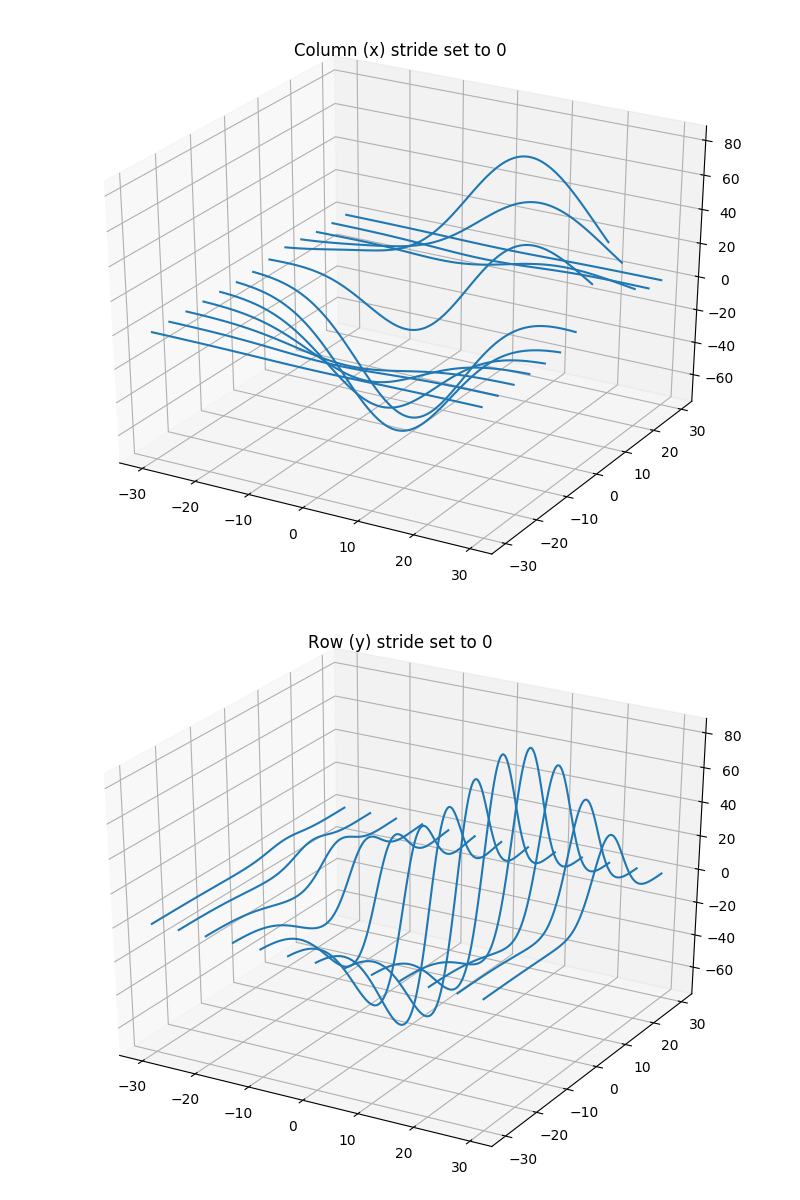Travis-CI:# 3D wireframe plots in one direction¶

Demonstrates that setting rstride or cstride to 0 causes wires to not be generated in the corresponding direction.```from mpl_toolkits.mplot3d import axes3d
import matplotlib.pyplot as plt

fig, [ax1, ax2] = plt.subplots(2, 1, figsize=(8, 12), subplot_kw={'projection': '3d'})

# Get the test data
X, Y, Z = axes3d.get_test_data(0.05)

# Give the first plot only wireframes of the type y = c
ax1.plot_wireframe(X, Y, Z, rstride=10, cstride=0)
ax1.set_title("Column (x) stride set to 0")

# Give the second plot only wireframes of the type x = c
ax2.plot_wireframe(X, Y, Z, rstride=0, cstride=10)
ax2.set_title("Row (y) stride set to 0")

plt.tight_layout()
plt.show()
```

Total running time of the script: ( 0 minutes 0.025 seconds)

Gallery generated by Sphinx-Gallery